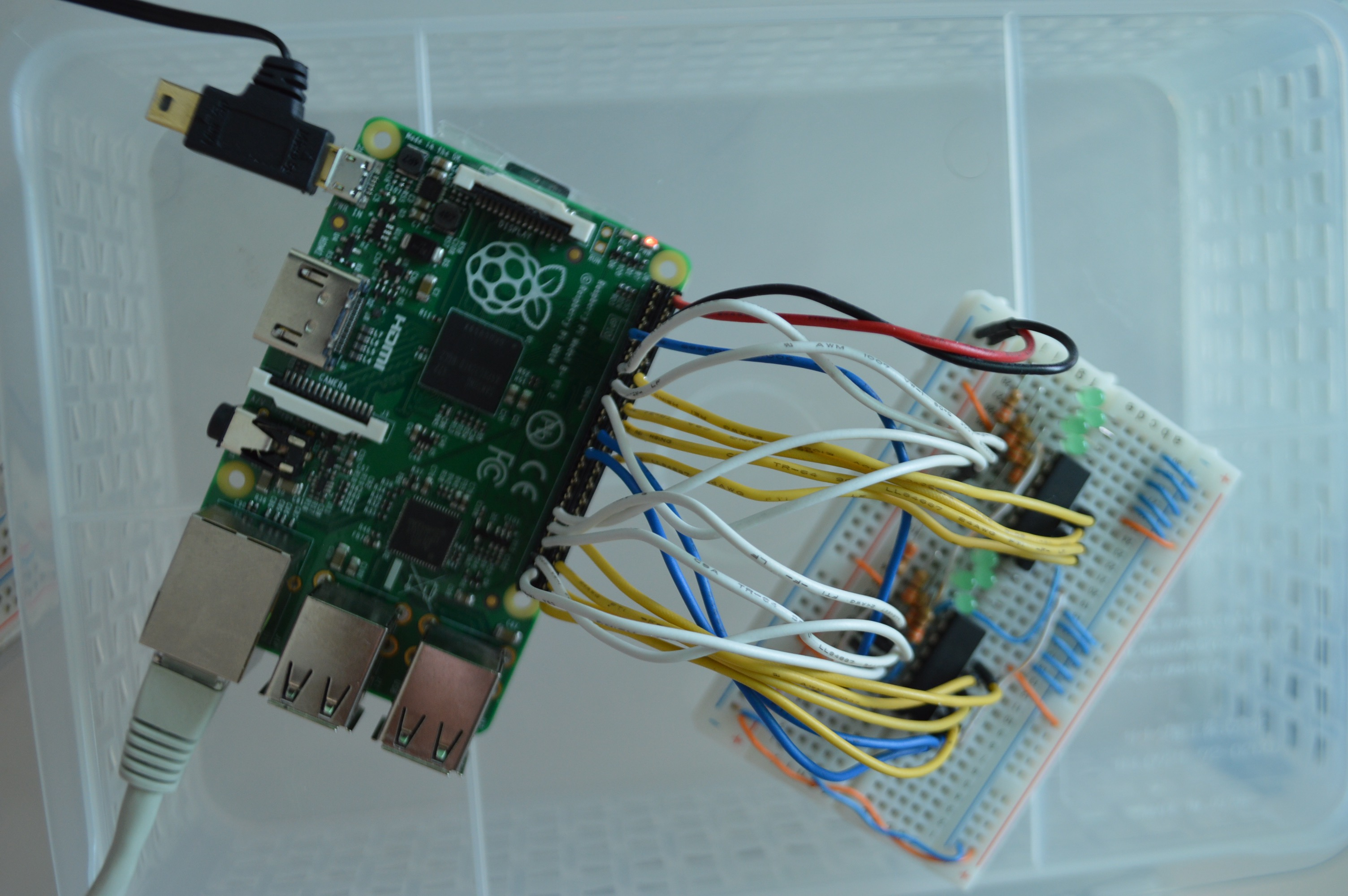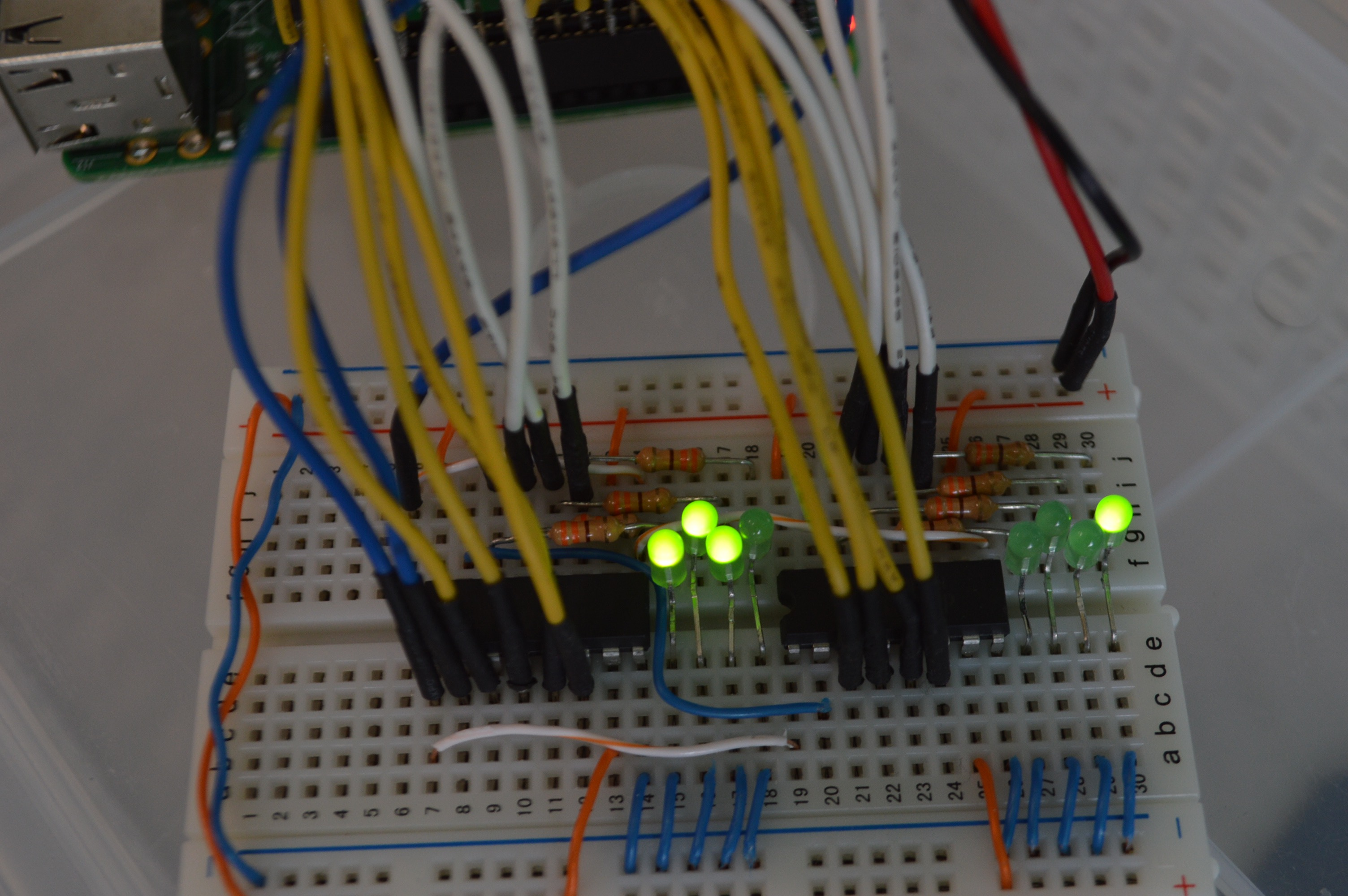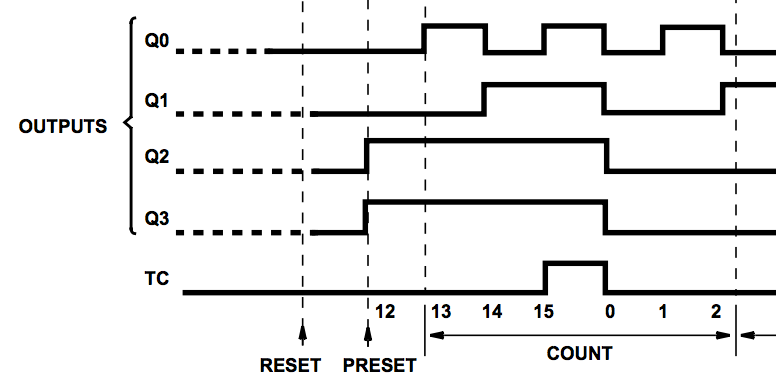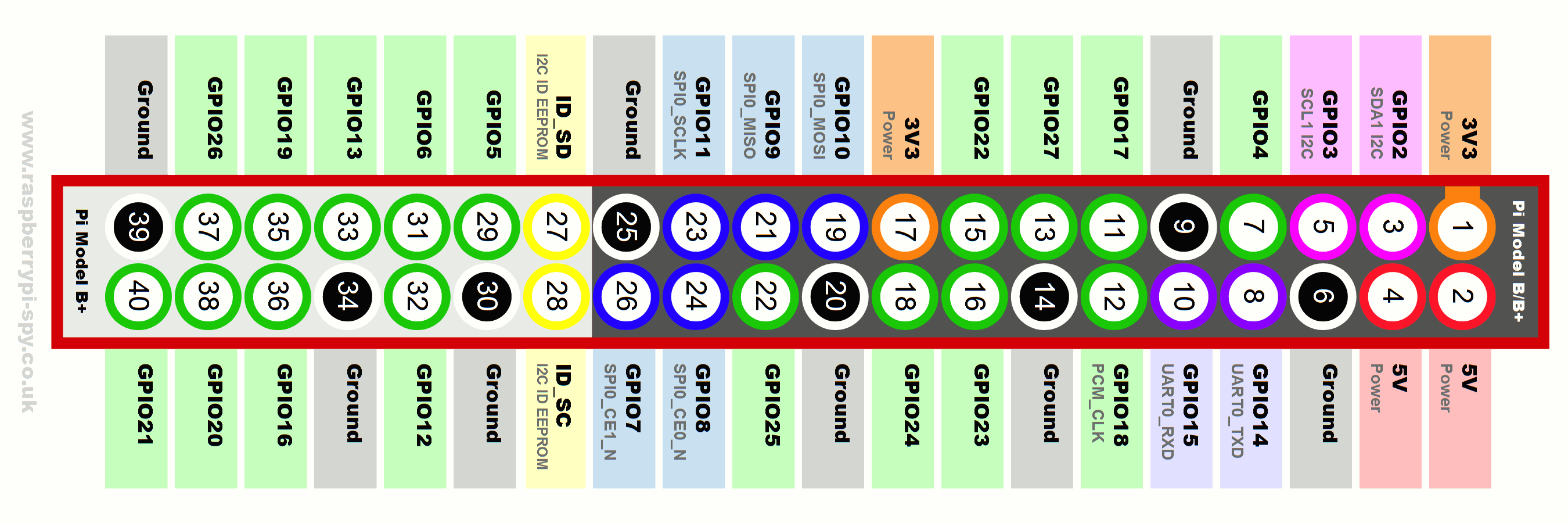# SECCON CTF 2015 - Hardware 2

We’ve found an encoder board using double 74HC161s along with a binary file.

## 1. Reverse hardwareAs in the problem, two ICs on the breadboard are 74HC161. According to the datasheet, it’s 4-bit counter. 4-bit counter is a circuit that changes its output 0000 -> 0001 -> 0010 -> 0011 -> … -> 1110 -> 1111 -> 0000, on every clock pulse.

The circuit implements one 8-bit counter using two 4-bit counters. Carry output(TC) of first counter is fed into clock(CP) of second counter. Thus, every time first counter overflows, second counter is incremented.Here’s a pitfall. The carry doesn’t work as expected. The counter is incremented when the clock pulses from low to high. According to the datasheet, the carry output pulses from low to high when the counter value changes from 14 to 15, not 15 to 0.

The breadboard is connected to a Raspberry Pi through its GPIO interface. We referred to GPIO pins layout to find which wire is connected to which interface.``````Output of first counter:
Q0 - gpio 26
Q1 - gpio 19
Q2 - gpio 13
Q3 - gpio 6
Output of second counter:
Q4 - gpio 5
Q5 - gpio 22
Q6 - gpio 27
Q7 - gpio 17
``````

## 2. Reverse Software

`gpio2.py` xors a file with circuit-generated values. The values are generated as follows:

1. Reads 8 bits from the counter in the order `Q0 Q7 Q6 Q5 Q4 Q3 Q2 Q1` (MSB to LSB).
2. Use this byte to xor one byte of input file.
3. Reset the circuit
4. Send clock pulse for (value + 3) times.

## 3. Decode

``````with open("encripted") as f:

v = 0
d = *len(e)

def inc(v):
lo = v&0xf
hi = ((v&0xf0) >> 4)
if lo == 0xe:
hi = (hi+1) & 0xf
lo = (lo+1) & 0xf
return lo | (hi<<4)

for i in range(len(d)):
v = ((v >> 1) | (v << 7)) & 0xff
d[i] = e[i] ^ v
n = v
v = 0
for _ in range(n+3):
v = inc(v)

d = ''.join(map(chr, d))
print d
``````

Decoded file is a gzip file.

``````\$ python decode.py > out
\$ file out
out: gzip compressed data, was "flag", from Unix, last modified: Mon Nov 30 13:52:44 2015
\$ cat out | gunzip
The flag is SECCON{7xgxUbQYixmiJAvtniHF}.
``````

#### Other posts (list)

SECCON CTF 2015 - Remote GDB
SECCON CTF 2015 - APK2
SECCON CTF 2015 - Hardware 2
안드로이드 앱 패킷 캡쳐하기
32C3 CTF - blobberry